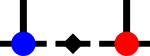SyTen## ◆ OffsetDenseTensor

template<Rank rank, typename Scalar = SDef>
 using syten::OffsetDenseTensor = typedef OffsetDenseTensorImpl::OffsetDenseTensor

A specialised offset dense tensor.

This tensor also stores a dense array of scalar coefficients like DenseTensor, but only for a certain part of its linear length.

That is, if tensor elements are identically zero for leading coordinates below or above some value, the offset dense tensor does not store them. As an example, consider the tensor $$T_{a,b,c,d}$$ with all dimensions equal to four. The tensor contains values if a is 2 and b is either 1 or 2. As a result, storing the elements of the tensor in a linear array gives us

| a = 0 | a = 1 | a = 2 | a = 3 |
+-----------------------+-----------------------+-----------------------+-----------------------+
| b=0 | b=1 | b=2 | b=3 | b=0 | b=1 | b=2 | b=3 | b=0 | b=1 | b=2 | b=3 | b=0 | b=2 | b=2 | b=3 |
+-----+-----+-----+-----+-----+-----+-----+-----+-----+-----+-----+-----+-----+-----+-----+-----+
| 0 | 0 | 0 | 0 | 0 | 0 | 0 | 0 | 0 | c,d | c,d | 0 | 0 | 0 | 0 | 0 |

where in the last line, each cell contains 16 elements but only those marked as c,d are actually non-zero. A standard dense tensor, however, still has to also store all 224 zero elements in addition to the 32 non-zero elements which is rather inefficient.

This type of tensor is generated during the product of standard dense tensors with rank-3 identity dense tensors, merging two tensor legs into one.